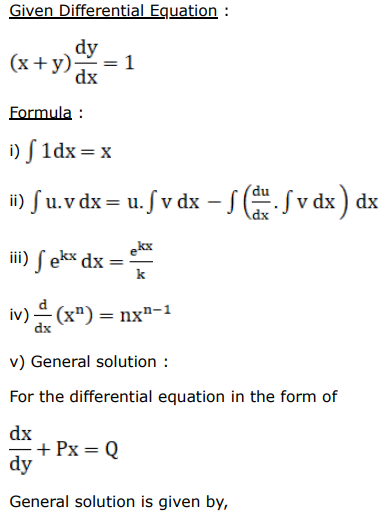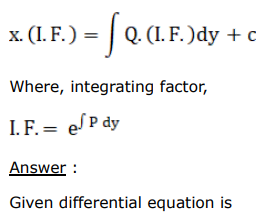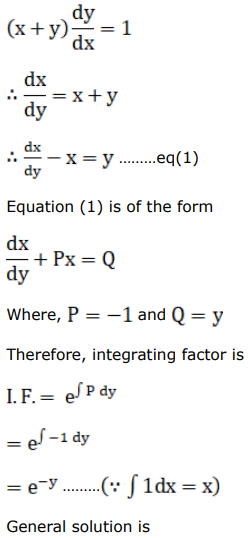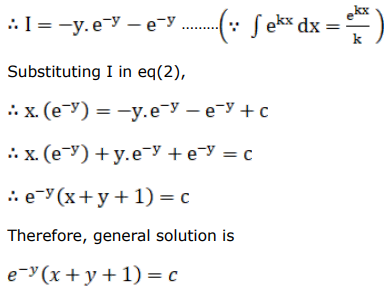# Find the general solution for each of the following differential equations.Question:

Find the general solution for each of the following differential equations.

$(x+y) \frac{d y}{d x}=1$

Solution: